# Skorokhod space

(diff) ← Older revision | Latest revision (diff) | Newer revision → (diff)
Jump to: navigation, search

Let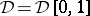be the space of real-valued functionsonthat are right-continuous and have left-hand limits, i.e.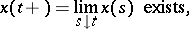(In probabilistic literature, such a function is also said to be a cadlag function, "cadlag" being an acronym for the French "continu à droite, limites à gauche" .) Introducing a norm onby setting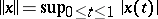, then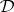becomes a Banach space, but it is easy to see that it is non-separable (cf. also Separable space). This non-separability causes well-known problems of measurability in the theory of weak convergence of measures on the space. To overcome this inconvenience, A.V. Skorokhod introduced a metric (and topology) under which the spacebecomes a separable metric space. Although the original metric introduced by Skorokhod has a drawback in the sense that the metric space obtained is not complete, it turned out (see [a6]) that it is possible to construct an equivalent metric (i.e., giving the same topology) under which the spacebecomes a separable and complete metric space (sometimes, for such a metric space the term Polish space is used). This metric is defined as follows.

Let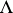denote the class of strictly increasing continuous mappings of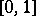onto itself. For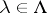, let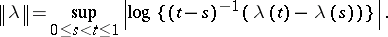Then for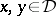one defines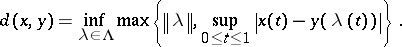The topology generated by this metric is called the Skorokhod topology and the complete separable metric spaceis called the Skorokhod space (cf. also Skorokhod topology). This space is very important in the theory of random processes (cf. also Stochastic process). The general theory of weak convergence of probability measures on metric spaces and, in particular, on the spaceis well developed. This theory was started in the fundamental papers [a4], [a6], [a10], [a11]. A well-known reference on these topics is [a1] (see also the updated second edition [a2]).

## Generalizations.

Several generalizations of the Skorokhod space are worth mentioning. Instead of real-valued functions onit is possible to consider functions defined on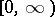and taking values in a metric space. The space of cadlag functions obtained in this way is denoted by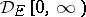and ifis a Polish space, then, with the appropriate topology, is also a Polish space, see [a7] and [a9], where these spaces are treated systematically.

Another generalization is obtained when the one-dimensional parameter(often regarded as "time" ) is replaced by multi-variate variable. Let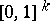denote unit cube in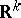. It is possible to introduce (see [a8], [a12]) the spaceof cadlag functions onand a Skorokhod topology on it. In [a3] one can find references and recent results on weak convergence of probability measures and, in particular, on the central limit theorem in this space.

In his fundamental paper [a11], Skorokhod introduced four topologies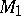,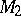,,; the topology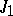became the most famous one and now bears his name (cf. also Skorokhod topology). At the end of the 1980s it was found that in certain problems the other topologies introduced by Skorokhod in the space of cadlag functions can be useful (see, for example, [a5], [a13], [a14]).

How to Cite This Entry:
Skorokhod space. Encyclopedia of Mathematics. URL: http://encyclopediaofmath.org/index.php?title=Skorokhod_space&oldid=13899
This article was adapted from an original article by Vygantas Paulauskas (originator), which appeared in Encyclopedia of Mathematics - ISBN 1402006098. See original article